﻿ Calculation of the Fatigue Life of a Dynamically Loaded ComponentPublications are Open
Access in this journal
Article Versions
Export Article
• Normal Style
• MLA Style
• APA Style
• Chicago Style
Research Article
Open Access Peer-reviewed

### Calculation of the Fatigue Life of a Dynamically Loaded Component

American Journal of Mechanical Engineering. 2017, 5(6), 252-256. DOI: 10.12691/ajme-5-6-4
Published online: December 15, 2017

### Abstract

The paper deals with fatigue calculations in the time and frequency domain that are focused on comparing the accuracy of results in applications with dynamically loaded components. Methods of numerical mechanics are used for the prediction of life compared to the results obtained by real component tests.

### 1. Introduction

The development of components is currently based on a large number of lengthy calculations and simulation strings that precede the prototype test 1. These strings include both static and dynamic analyses using various FEM simulations, MBS, NVH, modal analysis, temperature analysis, fluid flow, etc. Lastly, lifetime analysis is performed to find weak points in the structure. The decision whether and how the structure should be subjected to loading is based on distribution of damage. At present, we have two domains (sphere) in which lifetime analysis can be performed. The time domain is commonly used for fatigue life calculations. All input loads and output responses are described by time history. An alternative approach to evaluating fatigue life is frequency domain analysis. In this case, all loads and responses are represented as an energy dependence obtained at different frequencies.

### 2. Problem Formulation and Basic Equivalence

If the fatigue analysis should be performed in the time domain, it is necessary to known fatigue (Wohler) curves 2. Cycles and their stress value are calculated depending on chosen of a technique. The most used method is Rainflow method, and subsequent use of Palmgren-Miner rule makes it possible to estimate the lifetime of the part (mechanical system element). Estimating of time domain life is more accurate than the estimation done in the frequency domain, but it requires a much higher number of input data 3.

The first step in fatigue life calculation in the frequency domain is to estimate eigen frequencies that can be identified experimentally by measurement, or analytically using numerical methods such as finite element method. The next step is an estimate of the transient function describing stress or strain dependence on frequencies at the appropriate scale. This characteristic can be obtained before an experiment, an analytical or numerical solution. When using FEM, it is important to perform a dynamic frequency analysis that is the basis of modal analysis 4. Consequently, if the spectral power density and the transition function of the system are known, it is possible to define the spectral response of the load-bearing structure. For this analysis, one of the following probability models is required:

2.1. Dirlic

Dirlic’s model 5 is suitable for all kinds of signals.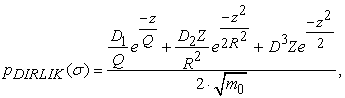(1)

where Z is the normalized amplitude, xm and R are the functions of the spectral moments m0 - m4. Subsequently D1, D2, D3, Q are parameters defined as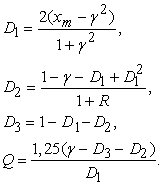(2)

Dirlic's formula for calculating the number of cycles to failure is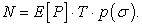(3)

where E[P] is the estimated number of peaks given by following equation: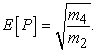(4)

The random of n-th spectral moment of the area under the PSD curve can be obtained from the equation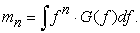(5)
2.2. Bendat - Narrowband Signals

Bendat's formula 6 for calculating the number of cycles to failure is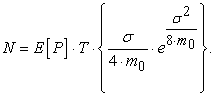(6)

Comparison of narrow band and broadband signal is shown in Figure 1.

• Figure 1. Bendat's method is conservative. 
2.3. Probability Density Function

Probability density function can be calculated from medium stress values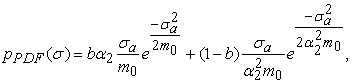(7)

where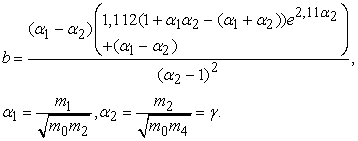(8)

For narrowband signals, it equals the unit of either α1 or α2, which results in a division as Rayleigh's distribution.

### 3. Experiment

3.1. Model Selection

The object of investigation was a holder of car component. The aim of analysis was to compare the results of FEM simulation with the results of real test. Figure 2 shows the holder assembly used in the practical experiment in which the holder with the component was excited by base vibrations corresponding to vehicle operation.

• Figure 2. The holder assembly used in experiment

In the simulation, the car component was replaced by an addition mass point attached to a green cover (Figure 3) with corresponding weight and inertia properties.

• Figure 3. The holder with additional weight

Specific values of inertia tensor components and mass matrix are listed in Table 1.

The modal analysis of the holder was performed for three different variants:

• model neglecting all inertia effects (mass uniformly distributed over the entire volume),

• model neglecting only deviations moments

• model including whole inertia tensor.

The aim was to assess the effect of the inertia tensor on the shift of eigenfrequencies and modal stress. The 1st and 2nd eigenmode can be seen in Figure 4. The results are listed in Table 2.

• Figure 4. Distribution of modal stress for 1st and 2th mode on model with a uniformly distributed mass over the entire volume

The value of the experimentally acquired the first eigen frequency was 26.14Hz. From Table 2, it can determine that the most accuracy model for the next lifetime analysis is the model UDM with neglecting all of the inertia effects.

3.2. The Power Spectral Density (PSD) Obtained from the Time History

For calculating of the lifetime in the frequency domain, it is necessary to obtain PSD (Power Spectral Density) from the time history of the measured excitation signal (Figure 5).

• Figure 5. Time dependence and PSD of excitation signal

The procedure for obtaining the PSD was as follows: In the first step, the acceleration time history was loaded from which a one-sided amplitude spectrum was obtained by means of Fast Fourier Transformation (FFT). For the FFT calculation, a Hanning window with 80% overlay and 2048 sampling was used, which basically correspond to the sampling frequency of the specified time signal. Amplitude correction was used to avoid uncertainties caused by using the Hanning window. It was necessary to divide the obtained PSD by 2 with regard to the mentioned unilateral amplitude spectrum.

When calculating the lifetime, it is possible to specify the spectrum of PSD in linear or logarithmic coordinates in many programs. The synthetic PSD that was also used to generate the time history had logarithmic coordinates. In contrast the PSD calculated from the accelerometer response time during the real test was obtained in linear coordinates. If the incorrect variant is used, the most overloaded segment may increase up to 50% on, what is also reflected in the lifetime calculation (Figure 6).

• Figure 6. PSD Comparison in Linear (True PSD) and Logarithmic (Synopsis PSD) Coordinates

### 4. Numerical Simulation

From the test result shown in Figure 7, it is possible to identify places where the holder has been damaged primarily.

• Figure 7. Result of the life of the holder
• Figure 8. Variants of numerical models for the holder

Three variants of numerical model were created in the attempt to increase lifetime of the holder (Figure 8). The first variant was without change (basic model). The second variant included the springs that should by represent a contact between the components. Their location is indicated in Figure 8 by a red circle. The springs were modeled as GAP elements with high stiffness in the direction of the contact and almost zero stiffness in the opposite direction. The last variant included the elastic layer representing a glue bonding of the components (pink area in Figure 8).

Two approaches were chosen for the time domain calculation: transient and quasi-static. Frequency analysis was used in the frequency domain. Figure 9 shows degree of failure for the most damaged node of the basic model and the modified model. Changed parameters of the modified model are shown in the Table 3. As can be seen in Figure 9, the damage for the modified model has been diminished.

• Figure 9. History of damage on model with fine mesh.
• Figure 10. Field of damage: a, b, transient analysis; c, d, frequency analysis; e, f, quasi-static analysis.

Figure 10 b, d, f, shows the fields of damage for the model with modified settings. It can be seen that a higher match with the real component test was achieved by changing these settings.

### 5. Results and Discussion

For both models, endurance was estimated at 06:34 [hour: min] in the frequency analysis, 02:39 [hour: min] in the transient analysis and 1662: 29 [hour: min] (about 70days) in the quasi-static analysis of lifetime. Compared to the real life of the holder 03:01 [hour: min], the most accurate method is the transient analysis, but this is the most conservative approach. The frequency lifetime analysis is also suitable for the types of tasks in which the dynamic behavior of the system is considered. It was shown that the extent and time of component damage are in good agreement with reality. On the other hand, the use of a quasi-static computational analysis for the lifetime of dynamically loaded components can by definitely excluded because this approach does not take into account the impact of dynamic effects on a structure. Since the results of the numerical analysis sufficiently correspond to the real test, it can be stated that the numerical model is sufficiently accurate.

### 6. Conclusion

The results of modal and lifetime analysis confirmed the correctness of the numerical model.

The most accurate results corresponding to the real behavior of the component loaded by the random stochastic signal were achieved by the transient approach in the time domain. The frequency approach to the fatigue life calculation has proven to be also sufficient. However, it is much faster compared to the transient approach.

From the calculated and measured data, it can be deduced that the most appropriate approach for calculating the dynamically stressed components is by means of frequency lifetime analysis.

### Acknowledgements

This research was supported by projects VEGA 1/0731/16 “Vývoj moderných numerických a experimentálnych metód analýzy mechanických sústav” and VEGA1/0393/14 “Analýza príčin porúch prvkov mechanických sústav kvantifikáciou polí deformácií a napätí”.

### References

  BOCKO P., Posudzovanie životnosti nosných konštrukcií dopravných strojov a zariadení s využitím numerických a experimentálnych metód. – Dissertation thesis. Košice: TUKE, 2006, 107. In article View Article  SVENSSON N., Fatigue Analysis with Loads from MBS - Master of Science Thesis. Stockholm, Sweden, 2015, 84. In article View Article  RUST H., Spectral Analysis of Stochastic Processes. Comorova, Romania, 2007, 76. In article View Article  HALFPENNY A., “A frequency domain approach for fatigue life estimation from FEA”, DAMAS99, Dublin, 9. In article View Article  TŮMA J., Zpracování signálů z mechanických systémů užitím FFT. Štramberk, 1997, 174. ISBN 80-901936-1 In article View Article  BISHOP N.W.M., SHERRATT F., Finite Element Based Fatigue Calculations. Farnharn, UK, 2000, 131. In article PubMed  NOVOVIČOVÁ J.: Pravděpodobnost a matematická statistika. Praha: ČVUT, 1999, 146. In article View ArticleThis work is licensed under a Creative Commons Attribution 4.0 International License. To view a copy of this license, visit http://creativecommons.org/licenses/by/4.0/